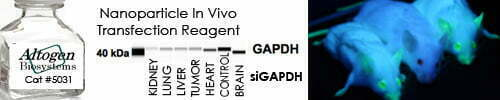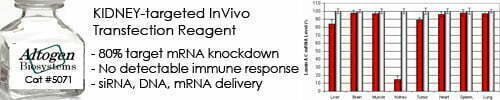••••Shop Products

# Percent Yield Calculator

A percent yield calculator is a tool that calculates the percentage yield of a chemical reaction based on the amount of product obtained in the reaction and the theoretical yield of the reaction. The percent yield is a measure of the efficiency of the reaction and is defined as the percentage of the theoretical yield that is actually obtained.

The formula for calculating percent yield is:

Percent Yield = (Actual Yield / Theoretical Yield) x 100%

Where:

• Actual Yield is the amount of product actually obtained in the reaction
• Theoretical Yield is the maximum amount of product that could be obtained based on stoichiometry calculations and assuming complete conversion of all reactants to products

To use a percent yield calculator, you need to input the values of actual yield and theoretical yield, and the calculator will calculate the corresponding percentage yield for you. The resulting percentage yield value can then be used to assess the efficiency of the chemical reaction and to identify potential sources of error or losses in the reaction.

Percent yield is an important concept in chemistry, where it is used to optimize reaction conditions, evaluate the purity of products, and determine the feasibility of chemical processes.

Percent Yield Calculator
Theoretical Yield:
g
Actual Yield:
g

Sorry, comments are closed for this post.